Olympiad Test Level 2: Addition and Subtraction - 1

# Olympiad Test Level 2: Addition and Subtraction - 1

Test Description

## 10 Questions MCQ Test Math Olympiad for Class 2 | Olympiad Test Level 2: Addition and Subtraction - 1

Olympiad Test Level 2: Addition and Subtraction - 1 for Class 2 2022 is part of Math Olympiad for Class 2 preparation. The Olympiad Test Level 2: Addition and Subtraction - 1 questions and answers have been prepared according to the Class 2 exam syllabus.The Olympiad Test Level 2: Addition and Subtraction - 1 MCQs are made for Class 2 2022 Exam. Find important definitions, questions, notes, meanings, examples, exercises, MCQs and online tests for Olympiad Test Level 2: Addition and Subtraction - 1 below.
Solutions of Olympiad Test Level 2: Addition and Subtraction - 1 questions in English are available as part of our Math Olympiad for Class 2 for Class 2 & Olympiad Test Level 2: Addition and Subtraction - 1 solutions in Hindi for Math Olympiad for Class 2 course. Download more important topics, notes, lectures and mock test series for Class 2 Exam by signing up for free. Attempt Olympiad Test Level 2: Addition and Subtraction - 1 | 10 questions in 20 minutes | Mock test for Class 2 preparation | Free important questions MCQ to study Math Olympiad for Class 2 for Class 2 Exam | Download free PDF with solutions
 1 Crore+ students have signed up on EduRev. Have you?
Olympiad Test Level 2: Addition and Subtraction - 1 - Question 1

### Which of the following is INCORRECT ?

Detailed Solution for Olympiad Test Level 2: Addition and Subtraction - 1 - Question 1

210 + 101 – 10 = 301
120 + 110 – 15 = 215
Thus, 301 > 215

Olympiad Test Level 2: Addition and Subtraction - 1 - Question 2

### Find the difference between two digit largest and two digit smallest number.

Detailed Solution for Olympiad Test Level 2: Addition and Subtraction - 1 - Question 2

2-digit largest number = 99
2-digit smallest number = 10
So, 99 – 10 = 89

Olympiad Test Level 2: Addition and Subtraction - 1 - Question 3

### There are 427 houses on one side of a road and 398 houses on the other side. How many houses are there along the road ?

Detailed Solution for Olympiad Test Level 2: Addition and Subtraction - 1 - Question 3

Total number of houses = 427 + 398 = 825

Olympiad Test Level 2: Addition and Subtraction - 1 - Question 4

Find the value of X + Y.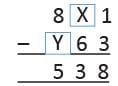Detailed Solution for Olympiad Test Level 2: Addition and Subtraction - 1 - Question 4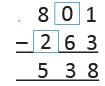So, X = 0 and Y = 2
Hence, X + Y = 0 + 2 = 2

Olympiad Test Level 2: Addition and Subtraction - 1 - Question 5

42 more than 42 and 8 more than 8 is :

Detailed Solution for Olympiad Test Level 2: Addition and Subtraction - 1 - Question 5

42 + 42 = 84
8 + 8 = 16
84 + 16 = 100

Olympiad Test Level 2: Addition and Subtraction - 1 - Question 6

The next number in the series is 34, 29, 24, ________.

Detailed Solution for Olympiad Test Level 2: Addition and Subtraction - 1 - Question 6

34, 29, 24, 19
34 – 29 = 5
29 – 24 = 5
24 – 19 = 5
As Difference between every number is 5, so the next number is 19.

Olympiad Test Level 2: Addition and Subtraction - 1 - Question 7

What will be the value of tens digit after the addition of numbers 58 and 24 ?

Detailed Solution for Olympiad Test Level 2: Addition and Subtraction - 1 - Question 7

58 + 24 = 8
8 tens

Olympiad Test Level 2: Addition and Subtraction - 1 - Question 8

Direction: Study the table carefully and answer the following questions.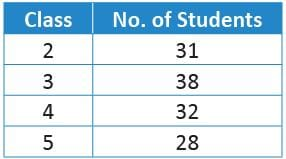Class 2 has how many students less than class 3 ?

Detailed Solution for Olympiad Test Level 2: Addition and Subtraction - 1 - Question 8

Class 3 – Class 2
= 38 – 31 = 7

Olympiad Test Level 2: Addition and Subtraction - 1 - Question 9

Which digit should come in the box so that following addition is correct ?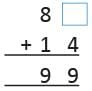Detailed Solution for Olympiad Test Level 2: Addition and Subtraction - 1 - Question 9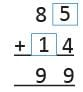Olympiad Test Level 2: Addition and Subtraction - 1 - Question 10

A coffee shop sells 160 small, 240 medium, 320 large cups of coffee in a week. How many total cups of coffee are sold in a week ?

Detailed Solution for Olympiad Test Level 2: Addition and Subtraction - 1 - Question 10

Total number of coffee cups = 160 + 240 + 320 = 720

## Math Olympiad for Class 2

72 tests
 Use Code STAYHOME200 and get INR 200 additional OFF Use Coupon Code
Information about Olympiad Test Level 2: Addition and Subtraction - 1 Page
In this test you can find the Exam questions for Olympiad Test Level 2: Addition and Subtraction - 1 solved & explained in the simplest way possible. Besides giving Questions and answers for Olympiad Test Level 2: Addition and Subtraction - 1, EduRev gives you an ample number of Online tests for practice

72 tests